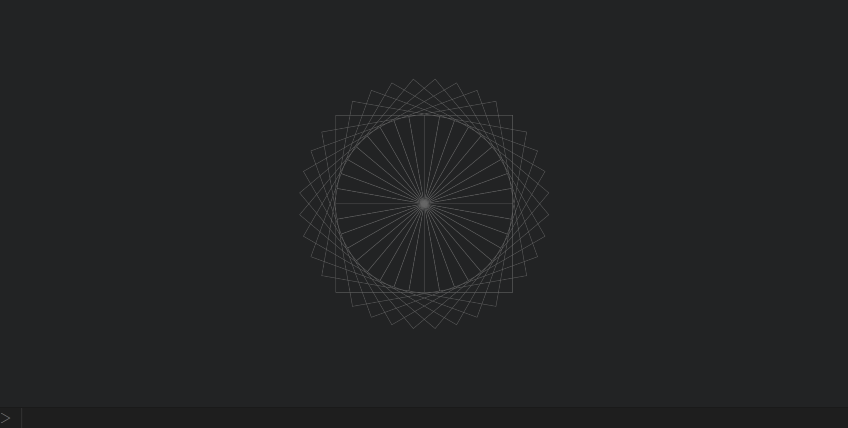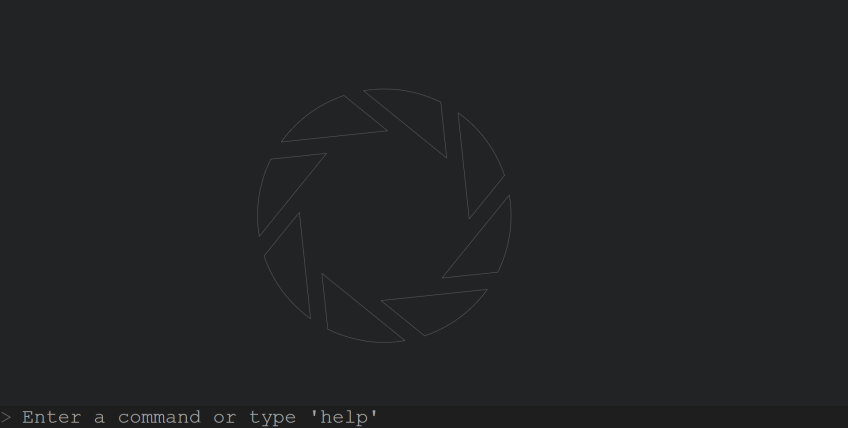# Turtle Console

You can view it here

## The commands are as follows:

• `go(amount)` -> moves turtle forward in the specified amount of pixels
• `move(amount)` -> moves turtle without drawing on the canvas
• `r(degrees)` / `right(degrees)` -> Turns the turtle right in the specified amount of degrees
• `l(degrees)` / `left(degrees)` -> Turns the turtle left in the specified amount of degrees
• `tran(x,y)` -> Changes the turtle's coordinates by adding x and y to current location
• `set(x,y)` -> Sets the absolute value of the turtles coordinates
• `color(#RRGGBB)` -> Sets the "pen" color
• `size(pixels)` -> Sets the line width
• `circ(radius)` -> Creates a circle with the turtle in the center

## Special commands:

• `v [variable name] = [value]` -> sets a variable
• `if ([condition]) ([code])` -> Executes specific code on a condition
• `for ([iterations]) ([code])` -> Executes a block of code for an amount of interations, specified by [iterations]

## Notes

In the "code box" or inside an if condition/for loop, every command must be trailed with a ;

## ExamplesThe following command will produce this output:
`for (36) (for (4) (l(90);go(100););l(10);)`Aperture Science! Credit goes to a redstone sheep

``````for (1) (
tran(0,300);
l(20);
for (8) (
for (12) (
go(15);
l(3);
);
l(130);
go(242);
l(135);
go(127);
l(59);
for (15) (
move(15);
l(3);
);
);
);``````